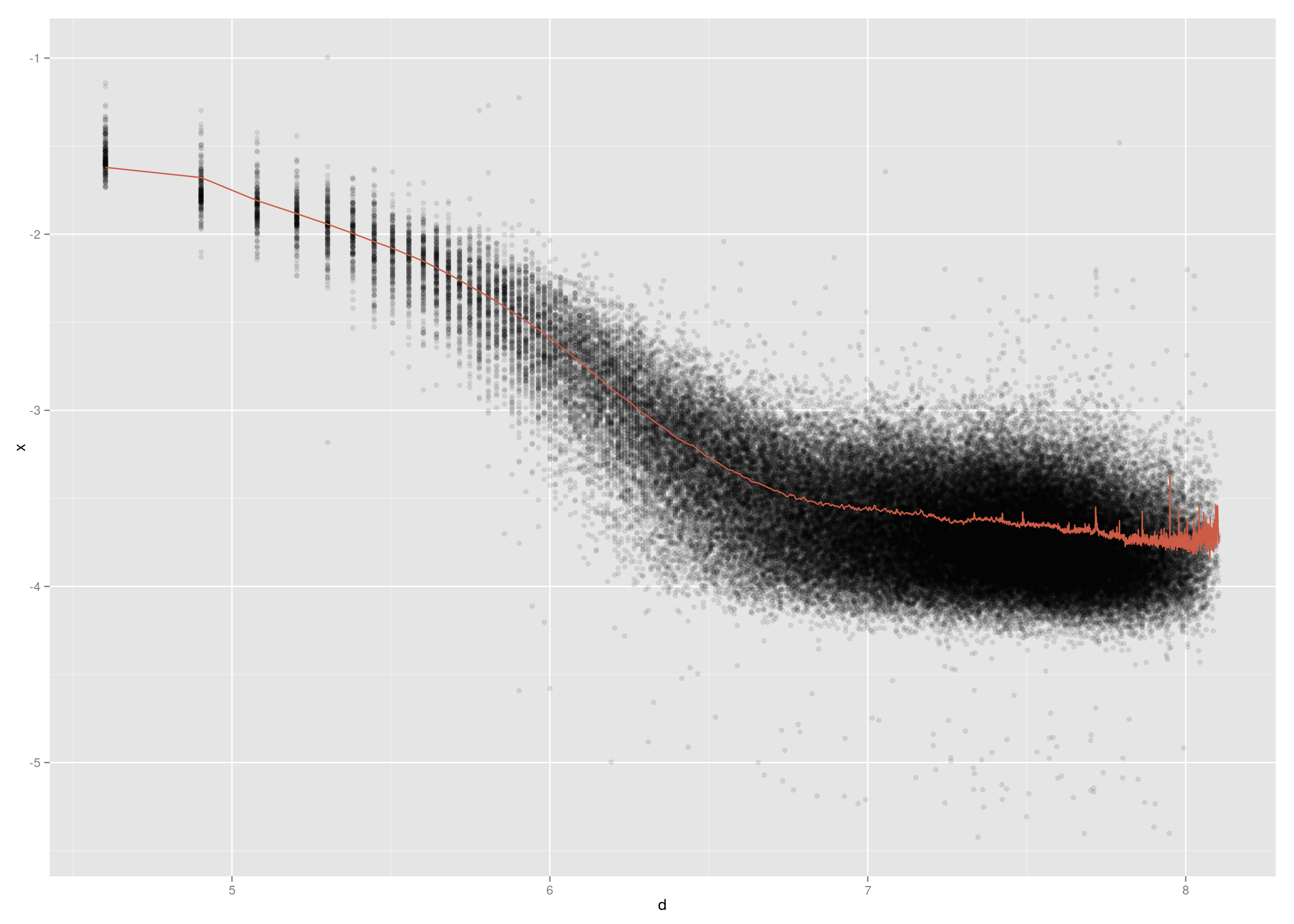Bernd Klaus

 European Molecular Biology Laboratory (EMBL), Heidelberg, Germany.

LAST UPDATE AT

Thu May 21 13:03:57 2015

Preparations

We first set global chunk options and load the neccessary packages.

library(BiocStyle)
library(rmarkdown)
library(geneplotter)
library(ggplot2)
library(plyr)
library(LSD)
library(gplots)
library(RColorBrewer)
library(stringr)
library(dplyr)
library(tidyr)
library(matrixStats)
library(genefilter)
library(locfit)
library(DESeq2)
library(LSD)
library(car)
library(vsn)

HiC data

We use ESC mouse data from Dixon et. al., 2012 processed by Aleksandra Pekowska.

The Ligation Frequency Matrices (LFM) obtained in an HiC experiment correspond to the number of filtered sequenced read pairs connecting two genomic intervals.

In order to reflect better physical proximity between sequences in the nucleus, these values need to be corrected for biases such as CG content bias, RF length biases and other unknown biases. To this end, an iterative correction (IC) was used on the LFM (Imakaev et al., 2012, Rao et al., 2014 ).

Instead of the original ICE (from Imakaev et. al.) algorithm, which is a variant of iterative proportional fitting (IPF) we have used the algorithm for IPF proposed and analyzed by Pukelsheim and Simeone since this has convergence garantuess and does not require prefiltering of the ligation frequencies.

We use these IC–corrected values as a starting point for the distance fit.

We have pooled results from chromosome 8 in ES cells, the bin size is 40kb bins.

The distance fit was performed using a simple moving average with window size of one bin so 40kb.

The observerd over expected values (OE) are on the log2 scale.

dataIC8 <- IC8[]
distanceFitMV <- IC8[]\$fit

##       i  j       x   i_pos   j_pos      d      OE OE_centered OE_centered_scaled
## 95   77 78 0.04307 3060000 3100000  40000  0.8466     1.23659            1.23659
## 973  77 79 0.02592 3060000 3140000  80000  0.3051     0.69513            0.69513
## 974  78 79 0.03425 3100000 3140000  40000  0.5157     0.90567            0.90567
## 2308 76 80 0.15529 3020000 3180000 160000  3.5701     3.96007            3.96007
## 2309 77 80 0.01399 3060000 3180000 120000 -0.1503     0.23966            0.23966
## 2310 78 80 0.01688 3100000 3180000  80000 -0.3141     0.07592            0.07592
##          fit distance
## [1,] 0.02395    40000
## [2,] 0.02098    80000
## [3,] 0.01553   120000
## [4,] 0.01308   160000
## [5,] 0.01140   200000
## [6,] 0.01011   240000

plot distance fit on a log10–log10 scale

Here we plot a sample of 100 000 of the log10–IC corrected values against the log10–distance. We see that the normalized interaction strenght decreases with distance.

dataGG <-  sample_n(dataIC8, 1e5)[, c("x","d")]
dataGG\$x <- log10(dataGG\$x)
dataGG\$d <- log10(dataGG\$d)
fitGG <- as.data.frame(distanceFitMV)
fitGG\$d <-  log10(fitGG\$distance)
fitGG\$fit <- log10(fitGG\$fit)
fitGG\$distance <- NULL

dataGG <- left_join(dataGG, fitGG)

(qplot(d, x, data = dataGG, alpha = I(1/10)) +
geom_line(aes(x = d, y = fit), color = I("coral3")))obtain distance fit via local regression

We use the package locfit to obtain a local regression fit. The predictors are wrapped in a call to ‘lp’, where we also define some tuning parameters. Unfortunately, there are not well explained in the help.

We also use loess as is done in the book. The locfit is plotted in blue and and the loess fit in magenta. We see that the loess and locfit functions give virtually identical results.

dataGG\$locFit  <- predict(locfit(x~lp(d, nn=0.5), data=dataGG), newdata = dataGG\$d)
dataGG\$loessFit <- predict(loess(x~d, span=0.5, data=dataGG))

(qplot(d, x, data = dataGG, alpha = I(1/10)) +
geom_line(aes(x = d, y = fit), color = I("coral3")) +
geom_line(aes(x = d, y = locFit), color = I("dodgerblue3")) +
geom_line(aes(x = d, y = loessFit), color = I("darkmagenta"))

)